# Dum-de-dum

## Recommended Posts

Ever had a period when you simply can't get into your work or things you need to do?

After coming back from Nozawa, I'm in one of those moods.

Nothing happening just can't click into gear and can't be bothered to... well do much at all really.

It would be ok if I was getting out and using the time constructively and having fun, but I'm just in one of those twiddling thumbs 'darui' modes.

Hmmmm.

##### Share on other sites

I've not been very productive this week at all - for work that is.

I have spent many hours online reading up on photo stuff and just totally become engrossed in that.

Getting a bit silly really, I need to snap out of that and get doing stuff.

Got a few things I need to catch up on now, which I'm not looking forward to.

##### Share on other sites

Problem 1.

72 / 4(6+3) = 2 or

72 / 4(6+3) = 117

Problem 2.

x = 0.99(recurring)

10x = 9.99(recurring)

10x-x = 9.99(recurring) - 0.99(recurring)

9x = 9

x = 1

x = 1 = 0.99(recurring)

1 = 0.99(recurring).

Problem 3.

ax/by = ax / by or (ax/b)y?

Actually none of these are problems. But math is fun. I want more of these stupid things.

(oooh, i should add that problem 3 isnt a problem, its a basic test that your math teacher might give you so that you understand the order of operations). It also conveniently shows why the answer to number IS 117 and not 2.

##### Share on other sites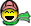I know what you mean though SKI.

Can't say I'm particularly productive right now.

Early spring blues!

##### Share on other sites

Problem 1.

72 / 4(6+3) = 2 or

72 / 4(6+3) = 117

Neither - the answer is 162 - 72 / 4 * (6+3) - BODMAS - Brackets over division, multiplication, addition and subtraction.

Problem 2.

x = 0.99(recurring)

10x = 9.99(recurring)

10x-x = 9.99(recurring) - 0.99(recurring)

9x = 9

x = 1

x = 1 = 0.99(recurring)

1 = 0.99(recurring).

Not really a problem is it? Seeing as you just mathematically proved it.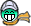Problem 3.

ax/by = ax / by or (ax/b)y?

ax / by as the second would be first do ax / b then divide that all by y.

##### Share on other sites

Two perfect logicians, S and P, are told that integers x and y have been chosen such that 1 < x < y and x+y < 100. S is given the value x+y and P is given the value xy. They then have the following conversation.

P: I cannot determine the two numbers.

S: I knew that.

P: Now I can determine them.

S: So can I.

Given that the above statements are true, what are the two numbers?
##### Share on other sites

"

"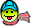Type entire question into google for rather elaborate solution (:

In a million years i wouldnt put that much effort into solving a puzzle. d:

Anyway, ax/by

questions about expressions similar to yours, from confused teachers

and students who have found that different books or teachers have

different answers, and even calculators disagree.

As written, your expression

ax/by

should be evaluated left to right: a times x, divided by b, times y.

The multiplication is not done before the division, but both are done

in the order they appear. Your first solution is right.

Some texts make a rule, as in your second solution, that

multiplication without a symbol ("implied multiplication") should be

done before any other operations in an expression, including "explicit

multiplication" using a symbol. Following this rule, you would

multiply a by x, then multiply b and y, then divide one by the other.

Some (probably most) texts don't mention such a rule - but some of

those may use it without saying so, which is far worse.

I don't know of a general rule among mathematicians that implied

multiplication should be done before explicit multiplication. As far

as I'm concerned, all multiplications fit in the same place in the

order of operations. It's not an unreasonable rule, though, since it

does seem that implied multiplication ties the operands together more

tightly, at least visually; but the idea of Order of Operations (or

precedence, as it is called in the computer world) is supposed to be

to ensure that everyone will interpret an otherwise ambiguous

expression the same way - so if some texts change the rules, or if

people do what feels natural, the purpose has been lost.

The problem here is that the expression looks as if it were meant to

be

ax

----

by

In the Dr. Math FAQ about writing math in e-mail, one of our

recommendations is to use parentheses wherever possible to avoid

ambiguity, even where the rules should make it clear, because it can

be easy to forget them in some situations:

So in e-mail we would write it like this:

ax/(by) or (ax/B)*y

depending on what is intended.

In my research for another Dr. Math "patient," I found that some

calculators have experimented with this rule. Calculators have

somewhat different needs than mathematicians, since they have to take

input linearly, one character after another, so they are forced to

make a decision about it. On the TI Web site I learned that they

deliberately put this "feature" into the TI 82, and then took it out

of the TI 83, probably because they decided it was not a standard rule

and would confuse people. Take a look at their explanation:

They also talk about a similar issue for exponentiation of the form

a^b^c, and give the same conclusion we give: always use parentheses

where a statement is ambiguous without special rules:

So to answer your question, I think both answers can be considered

right - which means, of course, that the question itself is wrong. I

prefer the standard way (your first answer) when talking to students,

unless their own text gives the "implicit multiplication first" rule;

but in practice if I came across that expression, I would probably

first check where it came from to see if I could tell what was

intended. The main lesson to learn is not which rule to follow, but

how to avoid ambiguity in what you write yourself. Don't give other

people this kind of trouble.

It helps explain the issue of number 3. Which in turn explains number 1 and why when we switch it all out into algebra it gets more straightforward.

a / b(f+d)

if we just go ahead and assume the uncontroversial thing and accept that we do f+d first (and call its product c), we get this:

a/bc

Which is a straight left to right operation...

a/b then multiply the product by c.

The end.

Or is it...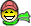Though i appreciate i did pull the solution from out of my ass to the actual puzzle posed. I got it in my head it was 13*9 for some bizarre reason (i had a brain fart and decided that 72/4 was actually 52/4. Just a human error cock up. 162 is right and what i meant to write.##### Share on other sites

Ippy and MiJ, you both need help, and I don't mean of the mathematical kind!!

Ever had a period when you simply can't get into your work or things you need to do?

Ya, that period is approaching 20 years.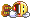##### Share on other sites

maths is FUN!

As i say, just break out any of those 3 in your class and have all your maths kids that hate english suddenly think youre the shit.##### Share on other sites

maths isn't FUN!

maths is shit.you typed too many words....I fixed it for you

##### Share on other sites

Two perfect logicians, S and P, are told that integers x and y have been chosen such that 1 < x < y and x+y < 100. S is given the value x+y and P is given the value xy. They then have the following conversation.

P: I cannot determine the two numbers.

S: I knew that.

P: Now I can determine them.

S: So can I.

Given that the above statements are true, what are the two numbers?

2 and 9?
##### Share on other sites

2 and 9?

Googling it, the answer turns out to be (won't spoil the surprise for others) a different pair of numbers, a pair that I had rejected last night for some reason. Oh well.

But the official answer was apparently found using a computer program (lazy!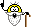), so I consider my answer gnarlier, albeit wrong.

##### Share on other sites

The funny thing M-O is that I also came up with two different numbers, think it was 2 & 7 but the kicker was that the answer can't be a prime number when either multiplied or added.

##### Share on other sites

Interesting thread this has turned into.....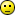........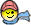##### Share on other sites

Ever had a period when you simply can't get into your work or things you need to do?

After coming back from Nozawa, I'm in one of those moods.

Nothing happening just can't click into gear and can't be bothered to... well do much at all really.

It would be ok if I was getting out and using the time constructively and having fun, but I'm just in one of those twiddling thumbs 'darui' modes.

Hmmmm.

Today is DEFINITELY one of them.

I have wasted pretty much it all doing sod all work.

Just a bit of pretending.

##### Share on other sites

Interesting thread this has turned into.............Too cerebral for you?##### Share on other sites

Ya...I can't keep up with all you mathamagicians. Cerebral is over-rated...much prefer da boobs and ass.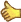##### Share on other sitesNot my cuppa, but just for you Chriselle!

##### Share on other sites##### Share on other sites
×
×
• Create New...